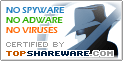Math Homework Maker 1.0

SNAPSHOT

Math Homework Maker,is a FREE software which can solve all your math homework

Windows AllPlatform :
\$0Price :
409 KBFile Size :
ScreenshotScreenshot :Popularity :The Math Homework Maker, is a FREE software which can solve all your Math homework.

If you are a pupil or a student, needing help with your math homework or just want to check them,
Then you must have this software.

The Math Homework Maker covers everything you need, from elementary school up to college.
Among other things it covers:

Conversion: Fraction, Improper Fractions, Decimal fractions.
Operators On Fractions:
[ add , subtraction, multiplication, division, bigger , equals ,smaller ] ,Common-Denominator, Reduction.
Areas and Perimeters: Quadrilaterals, Triangles, Circles.
Traffics and Percentages Problems.
Progression: Geometric Progression, Arithmetic Progression.
Triangles: The Law Of Sines, The Law Of Cosines.
Statistics Mean, Standard-Deviation ,Different.
Complex Numbers.
And Much More.

This software is so easy and friendly to use even if you are a parent who want to check your kids' homework, but have no knowledge at all in mathematics.